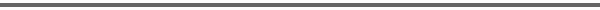Journal Home Page Complete Contentsof this Volume Previous Article Next Article Journal of Lie Theory 14 (2004), No. 2, 523--535 Copyright Heldermann Verlag 2004On Dimension Formulas for gl(m | n) Representations E. M. Moens Dept. of Applied Mathematics and Computer Science, Ghent University, Krijgslaan 281-S9, 9000 Gent, Belgium, ElsM.Moens@ugent.be Joris Van der Jeugt Dept. of Applied Mathematics and Computer Science, Ghent University, Krijgslaan 281-S9, 9000 Gent, Belgium, Joris.VanderJeugt@ugent.be[Abstract-pdf] We investigate new formulas for the dimension and superdimension of covariant representations $V_\lambda$ of the Lie superalgebra $\frak{gl}(m{|}n)$. The notion of $t$-dimension is introduced, where the parameter $t$ keeps track of the $\mathbb Z$-grading of $V_\lambda$. Thus when $t=1$, the $t$-dimension reduces to the ordinary dimension, and when $t=-1$ it reduces to the superdimension. An interesting formula for the $t$-dimension is derived from a recently obtained new formula for the supersymmetric Schur polynomial $s_\lambda(x/y)$, which yields the character of $V_\lambda$. It expresses the $t$-dimension as a simple determinant. For a special choice of $\lambda$, the new $t$-dimension formula gives rise to a Hankel determinant identity. [FullText-pdf (192 KB)] for subscribers only.